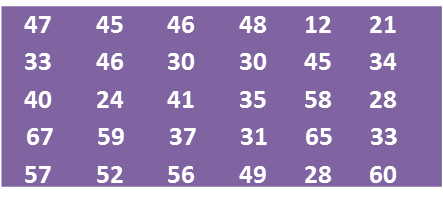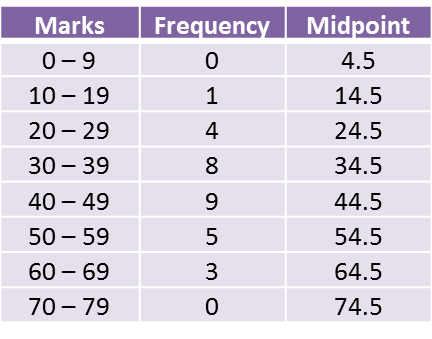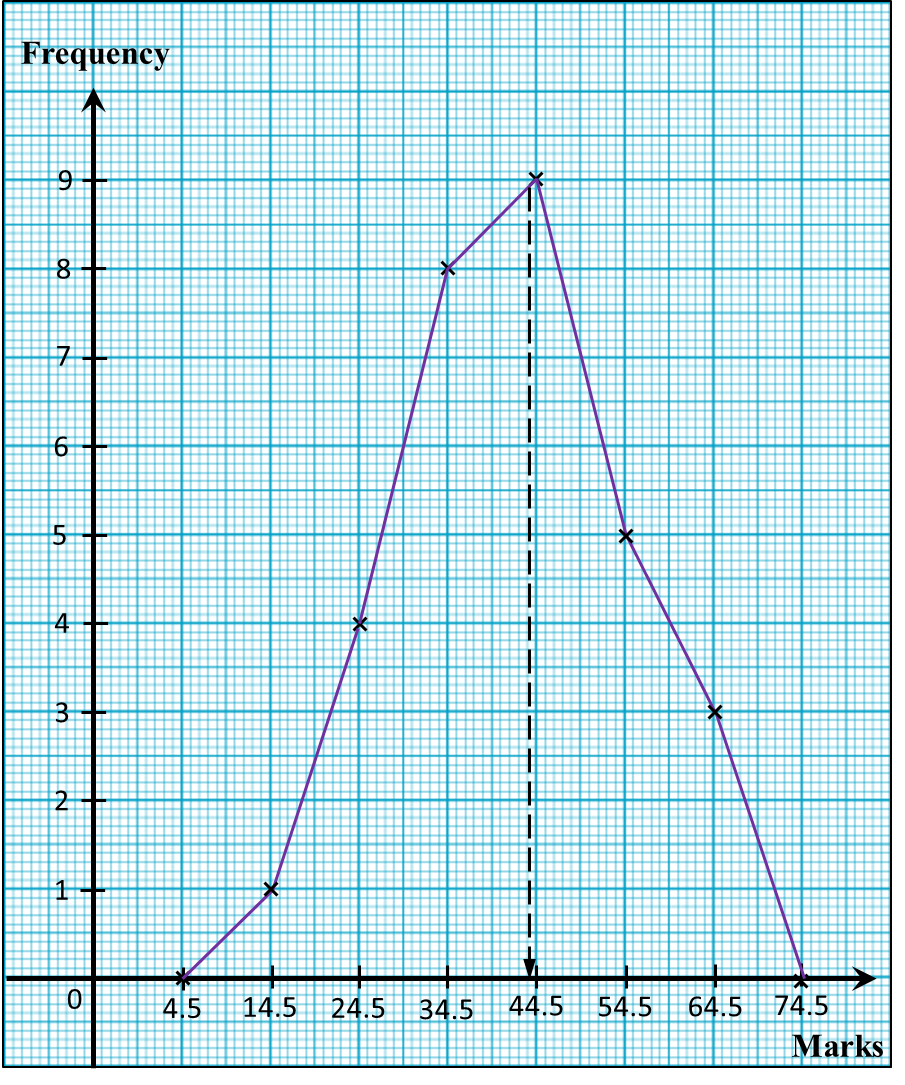# 6.8.2 Statistics, SPM Paper 2 (Long Questions)

Question 3:
The data below shows the mass, in kg, of 50 students.
 60    50    52   44   49   67   61   43    62   5347    54    58    60   56   41   69   45    51   63 56    58    59    47   48   45   65   47    58   51 64   62    60    56   56   61   64   57    62   64 64   46    48    61   60   63   53   43    63   62

(a) Copy and complete the table below based on the data above.
 Mass (kg) Midpoint Frequency 40 – 44 42 45 – 49
(b) Based on the completed table above,
(i) State the size of the class interval used in the table.
(ii) Calculate the estimated mean of the mass of the students.

For this part of the question, use graph paper.
(c) By using a scale of 2cm to 5 kg on the horizontal axis and 2cm to 2 students on the vertical axis, draw a frequency polygon for the data.

Solution:
(a)
 Mass (kg) Midpoint Frequency 40 – 44 42 4 45 – 49 47 9 50 – 54 52 7 55 – 59 57 9 60 – 64 62 18 65 – 69 67 3

(b)(i)
Size of class interval
= upper boundary – lower boundary
= 44.5 – 39.5
= 5

(b)(ii)
$\begin{array}{l}\text{Estimated mean =}\frac{42×4+47×9+52×7+57×9+62×18+67×3}{50}\\ \text{=}\frac{2785}{50}=55.7\end{array}$
(c)

Question 4:
Diagram below shows the marks obtained by a group of 30 students in a Science test.(a) Based on the data in diagram above, complete the table in the answer space.
(b) Based on the completed table in part (a), calculate the estimated mean mark of a student.
For this part of the question, use graph paper.
(c) By using a scale of 2 cm to 10 marks on the horizontal axis and 2 cm to 1 student on the vertical axis, draw a frequency polygon for the data.
(d) The passing mark for the test is 44. Using the frequency polygon drawn in part (c), find the number of students who passed the test.Solution:
(a)(b)(c)(d)
Number of students who passed the test
= 9 + 5 + 3
= 17